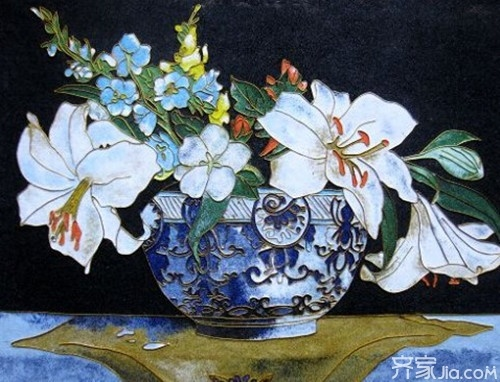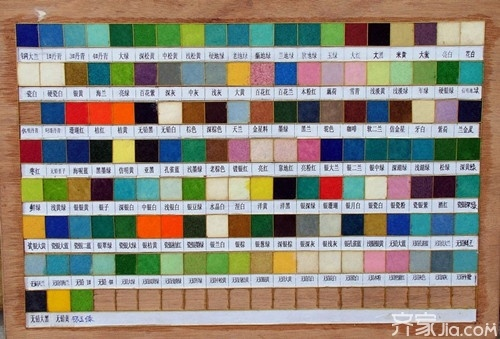|

# 景泰蓝工艺画厂家推荐 馈赠佳友家居装饰的不二选择

装饰画作为体现主人个性和内涵的一种室内装饰，为家居空间再添了几分灵性与情趣。装饰画是现代室内装修装饰的点睛之笔，各种风格的装饰画代表了不同的品位，中国的传统手工艺画景泰蓝工艺画近年来也是受到大家的欢迎。因为制作景泰蓝工艺画对制作人员有极大的技术要求，它都是纯手工制作，所以画作价格比较贵，但它的质量以及呈现出的画面都是最优的，景泰蓝工艺画是一种集艺术欣赏、收藏价值还有装饰作用一体的画作。市场反响特别好，那么，景泰蓝工艺画厂家有哪些？下面我为大家推荐几家不错的景泰蓝工艺画厂家，供消费者选购时参考！景泰蓝工艺画介绍

景泰蓝工艺画称彩沙画、金丝彩砂画、金丝彩釉画、金丝画、金丝沙画、景泰蓝装饰画、景泰蓝彩沙画、景泰蓝砂画等，是一道汉族传统工艺美术品，借鉴了传统景泰蓝工艺品中的嵌丝、点蓝技术，在平板媒介上用金属丝镶嵌出各种图型，景泰蓝工艺画用釉料、釉沙、彩沙等原料点蓝着色，最后将画面做抛光处理，使成品画面呈现水晶般透明、亮丽质感；也可以处理出磨砂、亚光、沙粒感、立体等多层次画面效果；还可以用无丝的沙画形式制作各种风景、山水等画面。

景泰蓝工艺画属于正做法工艺范畴，更适合制作花卉、人物、山水、花鸟等画面，可用丰富、自然、柔和的过渡色彩来表意。景泰蓝又名珐琅。是中国著名的手工艺 品。它起源于元朝，盛行于明朝景泰年间，距今有六百年历史。是皇宫中盛行的高雅艺术品，具有极高的欣赏价值、收藏价值和装饰效果。

景泰蓝工艺画是一种高档家居装饰品，分为无金丝和有金丝等多种制作方法,它融合各种工艺美术的手法与技法,由纯手工精制而成，以金属丝为线条，天然彩石为颜料，镶嵌成各种精美的图案。画片厚度在二毫米到几厘米之间，金属丝、彩石合成一体，画面平整光滑结实，彩石粒粒清晰可见。

景泰蓝工艺画具有类似黄金色（也有黑、白色）的金属丝条、粗犷的石头颗粒、亮丽的颜色、精制的做工，整幅画给人的感觉即古朴典雅，又高档华丽，是一种高档的家居饰品、馈赠礼品、手工艺品，具有鲜明的艺术特色，是文化创意艺术的顶峰之作，艺术界的奇迹,与景泰蓝略同，是工艺品与艺术品的结合，实用性与艺术价值宠于一体。景泰蓝工艺画材料

釉料是制作传统烧制景泰蓝工艺品的原料，它需要炉内经过高温烧制，出炉时是色块，然后用球磨机磨碎。

所以釉料外观是粉末状的，在做画前需要淘洗，淘洗的次数一般在5遍左右，洗出的水可到在容器中，待沉淀后，可用其色浆做画，这样既可不浪费还可使画面出现细腻的质感。最后将画面做抛光处理，使成品画面呈现水晶般透明、亮丽质感;也可以处理出磨砂、亚光、沙粒感、立体等多层次画面效果;还可以用无丝的沙画形式制作各种风景、山水等画面。

景泰蓝工艺画制作方法

景丝与釉的接合才会有中国景泰蓝，才会有景泰蓝工艺画，所以掐丝是重点．

丝掐得好不好，直接关系到画的整体效果．泰蓝工艺画在制作方面分为选图、描图、掐丝、点蓝、压模等几个步骤。其中选图、描图是前提，掐丝、点蓝是重点，压模是关键。

丝与釉的接合才会有中国景泰蓝，才会有景泰蓝工艺画，所以掐丝是重点．丝掐得好不好，直接关系到画的整体效果．粘丝一般过程

1.选好需要粘丝的线条，用胶水涂上

2.粘丝首先要缕丝，用剪刀和镊子都可以

3.检查丝的端口是否整齐

4.左手用镊子镊住丝端口正面的3/2放在线涂好胶线条的端口上

5.右手拿信丝的另一端弯出线条图形景泰蓝工艺画厂家推荐

北京汉艺煌景泰蓝工艺品有限公司创建于1973年至今已有近四十年专业制作景泰蓝的历史，经过几十年艰苦努力，公司已具备了雄厚的技术力量和多样的艺术风格。公司的主导产品“京景”牌景泰蓝，制作讲研，技艺精湛，数十次荣获国家、市级大奖。最近十几年，该公司在北京燕莎友谊商城、北京工艺美术大厦、北京市百货大楼等多家知名商场联营合作，批发业务也遍布全国各地。公司产品畅销于欧美市场。公司在北京市朝阳区东三环南路劲松桥设有专卖店。目前已成为国内最具实力的景泰蓝生产厂家。

重庆渝景工艺品有限公司是一家以生产景泰蓝工艺画原料与制作销售景泰蓝工艺画、景泰蓝工艺品、活性炭画于一体的企业，是中国西南部最具有影响力的景泰蓝系列生产销售公司。该公司对正做法和反做法两种工艺不断地改进和完善。改进后的正做法工艺在上色时更容易做出自然丰富的过渡色彩，并在最后的覆膜工艺中可达100%成功率。制作出来的景泰蓝画无论是装框的还是无框的都显得精致高档。反做法工艺是利用脱膜剂，在玻璃上做画，从而省去了传统做法中的描图工序，并采用颜料作画，降低了生产成本，最后成品画从玻璃上脱离下来，表面光亮如镜。正反两种工艺完全是纯手工制作，适用不同的产品要求，可以根据顾客要求的画面.色彩和成本选择不同的加工工艺。

北京市珐琅厂创建于1956年1月，郭沫若同志为其手书厂名， 是全国景泰蓝行业中唯一的一家中华老字号，生产经营景泰蓝规模最大专业企业，集景泰蓝工艺画产品研发、制作、商贸、旅游为一体，北京市重要外事接待单位，全国工业旅游示范点。其主导产品京珐牌景泰蓝销往全国及世界许多国家和地区, 集景泰蓝产品研发、制作、商贸、旅游为一体。

编辑总结：景泰蓝工艺画最大的特点就是能非常直观、一目了然的反映作画人内心深处的那种真善美和高雅的意境，那种对生活与艺术的追求以及作画人的心灵手巧等等。景泰蓝工艺画的适用范围也很广泛，作为装饰作用，如宾馆装饰、酒店装饰、优雅的咖啡厅装饰、酒楼装饰等等，还可以礼尚往来，作为馈赠亲友的高雅礼品。

`声明：本文由入驻焦点开放平台的作者撰写，除焦点官方账号外，观点仅代表作者本人，不代表焦点立场错误信息举报电话： 400-099-0099，邮箱：jubao@vip.sohu.com，或点此进行意见反馈，或点此进行举报投诉。`A B C D E F G H J K L M N P Q R S T W X Y Z
A - B - C - D - E
• A
• 鞍山
• 安庆
• 安阳
• 安顺
• 安康
• 澳门
• B
• 北京
• 保定
• 包头
• 巴彦淖尔
• 本溪
• 蚌埠
• 亳州
• 滨州
• 北海
• 百色
• 巴中
• 毕节
• 保山
• 宝鸡
• 白银
• 巴州
• C
• 承德
• 沧州
• 长治
• 赤峰
• 朝阳
• 长春
• 常州
• 滁州
• 池州
• 长沙
• 常德
• 郴州
• 潮州
• 崇左
• 重庆
• 成都
• 楚雄
• 昌都
• 慈溪
• 常熟
• D
• 大同
• 大连
• 丹东
• 大庆
• 东营
• 德州
• 东莞
• 德阳
• 达州
• 大理
• 德宏
• 定西
• 儋州
• 东平
• E
• 鄂尔多斯
• 鄂州
• 恩施
F - G - H - I - J
• F
• 抚顺
• 阜新
• 阜阳
• 福州
• 抚州
• 佛山
• 防城港
• G
• 赣州
• 广州
• 桂林
• 贵港
• 广元
• 广安
• 贵阳
• 固原
• H
• 邯郸
• 衡水
• 呼和浩特
• 呼伦贝尔
• 葫芦岛
• 哈尔滨
• 黑河
• 淮安
• 杭州
• 湖州
• 合肥
• 淮南
• 淮北
• 黄山
• 菏泽
• 鹤壁
• 黄石
• 黄冈
• 衡阳
• 怀化
• 惠州
• 河源
• 贺州
• 河池
• 海口
• 红河
• 汉中
• 海东
• 怀来
• I
• J
• 晋中
• 锦州
• 吉林
• 鸡西
• 佳木斯
• 嘉兴
• 金华
• 景德镇
• 九江
• 吉安
• 济南
• 济宁
• 焦作
• 荆门
• 荆州
• 江门
• 揭阳
• 金昌
• 酒泉
• 嘉峪关
K - L - M - N - P
• K
• 开封
• 昆明
• 昆山
• L
• 廊坊
• 临汾
• 辽阳
• 连云港
• 丽水
• 六安
• 龙岩
• 莱芜
• 临沂
• 聊城
• 洛阳
• 漯河
• 娄底
• 柳州
• 来宾
• 泸州
• 乐山
• 六盘水
• 丽江
• 临沧
• 拉萨
• 林芝
• 兰州
• 陇南
• M
• 牡丹江
• 马鞍山
• 茂名
• 梅州
• 绵阳
• 眉山
• N
• 南京
• 南通
• 宁波
• 南平
• 宁德
• 南昌
• 南阳
• 南宁
• 内江
• 南充
• P
• 盘锦
• 莆田
• 平顶山
• 濮阳
• 攀枝花
• 普洱
• 平凉
Q - R - S - T - W
• Q
• 秦皇岛
• 齐齐哈尔
• 衢州
• 泉州
• 青岛
• 清远
• 钦州
• 黔南
• 曲靖
• 庆阳
• R
• 日照
• 日喀则
• S
• 石家庄
• 沈阳
• 双鸭山
• 绥化
• 上海
• 苏州
• 宿迁
• 绍兴
• 宿州
• 三明
• 上饶
• 三门峡
• 商丘
• 十堰
• 随州
• 邵阳
• 韶关
• 深圳
• 汕头
• 汕尾
• 三亚
• 三沙
• 遂宁
• 山南
• 商洛
• 石嘴山
• T
• 天津
• 唐山
• 太原
• 通辽
• 铁岭
• 泰州
• 台州
• 铜陵
• 泰安
• 铜仁
• 铜川
• 天水
• 天门
• W
• 乌海
• 乌兰察布
• 无锡
• 温州
• 芜湖
• 潍坊
• 威海
• 武汉
• 梧州
• 渭南
• 武威
• 吴忠
• 乌鲁木齐
X - Y - Z
• X
• 邢台
• 徐州
• 宣城
• 厦门
• 新乡
• 许昌
• 信阳
• 襄阳
• 孝感
• 咸宁
• 湘潭
• 湘西
• 西双版纳
• 西安
• 咸阳
• 西宁
• 仙桃
• 西昌
• Y
• 运城
• 营口
• 盐城
• 扬州
• 鹰潭
• 宜春
• 烟台
• 宜昌
• 岳阳
• 益阳
• 永州
• 阳江
• 云浮
• 玉林
• 宜宾
• 雅安
• 玉溪
• 延安
• 榆林
• 银川
• Z
• 张家口
• 镇江
• 舟山
• 漳州
• 淄博
• 枣庄
• 郑州
• 周口
• 驻马店
• 株洲
• 张家界
• 珠海
• 湛江
• 肇庆
• 中山
• 自贡
• 资阳
• 遵义
• 昭通
• 张掖
• 中卫

1室1厅1厨1卫1阳台

1
2
3
4
5

0
1
2

1

1

0
1
2
3报名成功，资料已提交审核A B C D E F G H J K L M N P Q R S T W X Y Z
A - B - C - D - E
• A
• 鞍山
• 安庆
• 安阳
• 安顺
• 安康
• 澳门
• B
• 北京
• 保定
• 包头
• 巴彦淖尔
• 本溪
• 蚌埠
• 亳州
• 滨州
• 北海
• 百色
• 巴中
• 毕节
• 保山
• 宝鸡
• 白银
• 巴州
• C
• 承德
• 沧州
• 长治
• 赤峰
• 朝阳
• 长春
• 常州
• 滁州
• 池州
• 长沙
• 常德
• 郴州
• 潮州
• 崇左
• 重庆
• 成都
• 楚雄
• 昌都
• 慈溪
• 常熟
• D
• 大同
• 大连
• 丹东
• 大庆
• 东营
• 德州
• 东莞
• 德阳
• 达州
• 大理
• 德宏
• 定西
• 儋州
• 东平
• E
• 鄂尔多斯
• 鄂州
• 恩施
F - G - H - I - J
• F
• 抚顺
• 阜新
• 阜阳
• 福州
• 抚州
• 佛山
• 防城港
• G
• 赣州
• 广州
• 桂林
• 贵港
• 广元
• 广安
• 贵阳
• 固原
• H
• 邯郸
• 衡水
• 呼和浩特
• 呼伦贝尔
• 葫芦岛
• 哈尔滨
• 黑河
• 淮安
• 杭州
• 湖州
• 合肥
• 淮南
• 淮北
• 黄山
• 菏泽
• 鹤壁
• 黄石
• 黄冈
• 衡阳
• 怀化
• 惠州
• 河源
• 贺州
• 河池
• 海口
• 红河
• 汉中
• 海东
• 怀来
• I
• J
• 晋中
• 锦州
• 吉林
• 鸡西
• 佳木斯
• 嘉兴
• 金华
• 景德镇
• 九江
• 吉安
• 济南
• 济宁
• 焦作
• 荆门
• 荆州
• 江门
• 揭阳
• 金昌
• 酒泉
• 嘉峪关
K - L - M - N - P
• K
• 开封
• 昆明
• 昆山
• L
• 廊坊
• 临汾
• 辽阳
• 连云港
• 丽水
• 六安
• 龙岩
• 莱芜
• 临沂
• 聊城
• 洛阳
• 漯河
• 娄底
• 柳州
• 来宾
• 泸州
• 乐山
• 六盘水
• 丽江
• 临沧
• 拉萨
• 林芝
• 兰州
• 陇南
• M
• 牡丹江
• 马鞍山
• 茂名
• 梅州
• 绵阳
• 眉山
• N
• 南京
• 南通
• 宁波
• 南平
• 宁德
• 南昌
• 南阳
• 南宁
• 内江
• 南充
• P
• 盘锦
• 莆田
• 平顶山
• 濮阳
• 攀枝花
• 普洱
• 平凉
Q - R - S - T - W
• Q
• 秦皇岛
• 齐齐哈尔
• 衢州
• 泉州
• 青岛
• 清远
• 钦州
• 黔南
• 曲靖
• 庆阳
• R
• 日照
• 日喀则
• S
• 石家庄
• 沈阳
• 双鸭山
• 绥化
• 上海
• 苏州
• 宿迁
• 绍兴
• 宿州
• 三明
• 上饶
• 三门峡
• 商丘
• 十堰
• 随州
• 邵阳
• 韶关
• 深圳
• 汕头
• 汕尾
• 三亚
• 三沙
• 遂宁
• 山南
• 商洛
• 石嘴山
• T
• 天津
• 唐山
• 太原
• 通辽
• 铁岭
• 泰州
• 台州
• 铜陵
• 泰安
• 铜仁
• 铜川
• 天水
• 天门
• W
• 乌海
• 乌兰察布
• 无锡
• 温州
• 芜湖
• 潍坊
• 威海
• 武汉
• 梧州
• 渭南
• 武威
• 吴忠
• 乌鲁木齐
X - Y - Z
• X
• 邢台
• 徐州
• 宣城
• 厦门
• 新乡
• 许昌
• 信阳
• 襄阳
• 孝感
• 咸宁
• 湘潭
• 湘西
• 西双版纳
• 西安
• 咸阳
• 西宁
• 仙桃
• 西昌
• Y
• 运城
• 营口
• 盐城
• 扬州
• 鹰潭
• 宜春
• 烟台
• 宜昌
• 岳阳
• 益阳
• 永州
• 阳江
• 云浮
• 玉林
• 宜宾
• 雅安
• 玉溪
• 延安
• 榆林
• 银川
• Z
• 张家口
• 镇江
• 舟山
• 漳州
• 淄博
• 枣庄
• 郑州
• 周口
• 驻马店
• 株洲
• 张家界
• 珠海
• 湛江
• 肇庆
• 中山
• 自贡
• 资阳
• 遵义
• 昭通
• 张掖
• 中卫• 手机• 分享
• 设计
免费设计
• 计算器
装修计算器
• 入驻
合作入驻
• 联系
联系我们
• 置顶
返回顶部